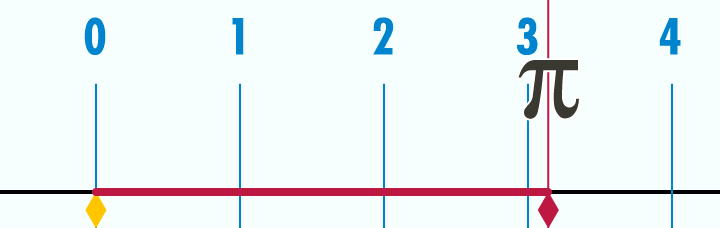## How to Calculate the Area of a Circle

Last week, we have discussed how to calculate the circumference of a circle. In this post, we learn how to calculate the area of a circle. The area of a circle which we will denote by$A$ is equal to the product of$\pi$ and the square of its radius$r$. Putting it in equation, we have$A = \pi r^2$.

In the examinations, the value of$\pi$ is specified. They usually use$3.14$,$3.1416$ or$\frac{22}{7}$.

If you can recall, the radius is the segment from the center to the point on the circle as shown below. The radius is half the diameter. The diameter is the longest segment that you can draw from one point on the circle to another. It always passes through the center.

Note: We also use the term radius to refer to the length of the radius and diameter as the length of the diameter.   » Read more

## The Operations on Integers Series

In the previous post, I have mentioned that it is important for you to master basic mathematics. One of the basic skills that you should master is operations on integers. Luckily, I have finished writing all the tutorials and reviewers for all the operations on integers.  They are listed below.

Good luck and have fun. I will be posting the quizzes and PDF exercises soon, so keep posted.

## It’s 3 Months Before the Next Exam! 4 Things To do

Yes, it’s three months before the next 2014 Civil Service Exam.If the original schedule pushes through, then the next exam will be on October 26th, exactly 3 months from now.

I think it is the perfect time for you to start your review seriously. Here are some of the things that you can do.

1.) Know the scope of the exam.

The exam is mainly composed of English and Mathematics. The details of the scope of the Civil Service Professional Exam and Sub-professional Exam can be found here. Focus on these types of exams, read, and practice everyday.  » Read more

## When to Use the Personal Pronouns I and Me

When do we use the personal pronouns I or me?

We use the word “I” when the pronoun is the subject of the verb. Meanwhile, we use the “me” if we are referring to the object of the verb.

Quick and dirty tip!

If you are confused, try to temporarily take out the other subject and read out loud. If it sounds nice, then it should be the correct one.

Example: (I, me) will go to Dumaguete with Jhody.

1. I will go to will go to Dumaguete with Jhody.
2. Me will go to Dumaguete with Jhody.  » Read more

## How to Calculate the Circumference of a Circle

In the previous post, we have learned about the basic terminologies about circles. We continue this series by understanding the meaning of circumference of a circle. The circumference of a circle is basically the distance around the circle itself. If you want to find the circumference of a can, for example, you can get a measuring tape and wrap around it.

The animation below shows, the meaning of circumference. As we can see, the circle with diameter 1 has circumference$\pi$ or approximately$3.14$.Note: If you want to know where$\pi$ came from, read Calculating the Value of Pi.

Example 1

What is the perimeter of a circle with diameter 1 unit?  » Read more

## Introduction to the Basic Concepts of Circles

The Civil Service Exams also contain geometry problems, and so far, our discussions are mostly algebra problems. In this new series of posts, we will discuss how to solve geometry and measurement problems particularly about circles. However, before we start solving problems, let us first discuss the basic terminologies about circles.

A circle is a set of points equidistant to a point called the center of the circle. As I go around to give trainings and lectures , I usually hear the wrong definition below. I am not sure where this definition originated, but this is wrong.  » Read more

## Grammar Tutorial: Subject-Verb Agreement

Subject-verb agreement:

If the subject is singular the verb should have “s” at the end. If the subject is plural there is no “s” at the end of the verb, except for the word “I” which always take the plural form of the verb.

Example: Jenna writes a poem.

In this sentence, the subject Jenna is singular, so the verb writes has “s” at the end.

Example: We visit our grandparents every Sunday.

In this sentence, the subject we is plural, so the verb visit has no “s.”

## Grammar and Correct Usage Quiz 7

This is the seventh part of the Grammar and Correct Usage Quiz Series.

Grammar and Correct Usage Quiz 7

Fill in the blanks with the most appropriate word.

1. The group insisted on an investigation of ___ member’s dismissal and threatened to sue the company.

a.) its
b.) their
c.) there
d.) it’s